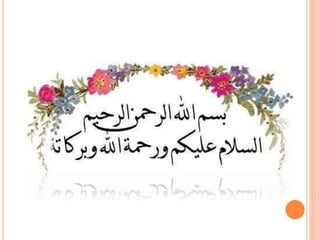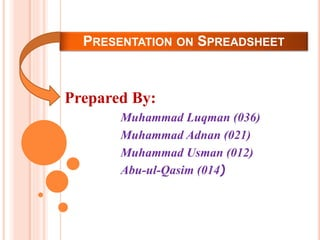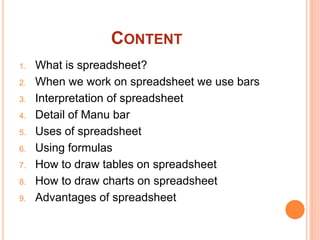Successfully reported this slideshow.

# Final Presentation on Spreadsheet Computer by Luqman×

1 of 17
1 of 17

# Final Presentation on Spreadsheet Computer by Luqman

Sargodha
UOS Presentation 2016

Sargodha
UOS Presentation 2016

### Final Presentation on Spreadsheet Computer by Luqman

2. 2. CONTENT 1. What is spreadsheet? 2. When we work on spreadsheet we use bars 3. Interpretation of spreadsheet 4. Detail of Manu bar 5. Uses of spreadsheet 6. Using formulas 7. How to draw tables on spreadsheet 8. How to draw charts on spreadsheet 9. Advantages of spreadsheet
3. 3. WHAT IS SPREADSHEET? An electronic document in which data is arranged in the rows and columns of a grid and can be manipulated and used in calculations.
4. 4. WHEN WE WORK ON S.S WE USE BARS
5. 5. WHEN WE WORK ON S.S WE USE BARS
6. 6. DETAIL MENU BAR  File Save-Save as-New-Print-Close-Exit  Home Clipboard-Font-Alignment-Number-Style- Cell-Editing  Insert Table-Chart-Symbol-Text  Page Layout Theme-Page Setup-Arrange-Scale-Sheet
7. 7. CONTINUE……….  Formulas Calculations-Formula Appling-Defined Name  Data Data Tools-Get External Data-Connection  Review Spellings-Language-Comments-Changes  View Window-Zoom-Show-Workbook Views
8. 8. USES OF SPREADSHEET  Modeling and Planning  Business Accounts and Budgeting  Calculations e.g. Adding, Subtracting, etc.  Creating Graphs e.g. bar chart, pie chart  Collect data from different sources e.g. phone number, prices  Explore and interpret data in order to draw conclusions for business
9. 9. USING FORMULAS BASIC MATH  To add up the Total  To find the change /difference  Percent change  Percent of total  Find average  Find median  For maximum value  Find minimum value =sum(cell range) =New value – old value =(new value-old value)/old value =part/hole =average(B2:B9) =median(cell rang) =max(cell range) =Min(cell range)
10. 10.  For 1st word capitalized  For each word capitalized  For each word small =proper(cell) =upper(cell) =lower(cell) SIMPLE FORMATTING TRICKS
11. 11. PULLING THINGS APART  For left side the text  For center of the Text  For right side the text  Find the text which you want =left(cell range,4) =mid(cell range,9,4) =right(cell range,3) =search(“,”,cell range)
12. 12. PUTTING THINGS TOGETHER DEALING DATE  If you wanted to put all of the pieces of each cell address  If you want to find year  If you want to find day  If you want to find month =Concatenate(A2,””,B2,”” ,C2,””,D2) =year(cell range) =day(cell range) =month(cell range)
13. 13. HOW TO DRAW TABLE o First of all you enter the data on spreadsheet o Select the data on spreadsheet o Than you click on the insert option o Click on table Example
14. 14. HOW WE DRAW CHART? o Inter the data in spreadsheet o Select the data o Click on menu bar (insert option) o Click the chart box(Column, Line & Pie etc) Example 0 10 20 30 40 50 60 70 80 90 100 BCTF16E036 BCTF16M021 BCTF16M012 BCTF16M014 M.Luqman M.Adnan M.Usman Abu-ul-Qasim 1 2 3 4 No. No.
15. 15. ADVANTAGES OF SPREADSHEET  Calculations are correct  Calculations are completed automatically  Information is organized and easy to access  Information is easy to edit if a mistake has been made by retyping or using ‘undo’  Data can be easily sorted and filtered  Data can be quickly analyzed  Reports can be made more visual by using charts and graphs